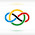### 2016 EGMO #2

Let $ABCD$ be a cyclic quadrilateral, and let diagonals $AC$ and $BD$ intersect at $X$.Let $C_1,D_1$ and $M$ be the midpoints of segments $CX,DX$ and $CD$, respectively. Lines $AD_1$ and $BC_1$ intersect at $Y$, and line $MY$ intersects diagonals $AC$ and $BD$ at different points $E$ and $F$, respectively. Prove that line $XY$ is tangent to the circle through $E,F$ and $X$.

Because $DD_1=D_1X$ and $CC_1=C_1X$ we obtain $\triangle XD_1C_1 \sim \triangle XDC$. So $\angle D_1C_1X= \angle DCX= \angle XBA$. Thus $D_1C_1BA$ is cyclic implying $\angle D_1AX= \angle XBC_1$. Because $DM=MC$ and $XC_1=C_1C$ we have $\triangle CMC_1 \sim \triangle CDX$. So, $C_1M= \frac{DX}{2} = D_1X$ and $MC_1 \parallel DB$. Now, because $\triangle YD_1C_1 \sim \triangle YBA$ and $\triangle D_1C_1X \sim \triangle ABX$, we get$\frac{YC_1}{YA} = \frac{D_1C_1}{AB} = \frac{D_1X}{XA} = \frac{MC_1}{XA}$. So, finally, we have $\triangle YXA \sim \triangle YMC_1 \sim \triangle YFB$ which implies $\angle YXE= \angle EFX$, as desired. $\square$

1.mahaler12/31/2021

Cool solution!

2.@mahaler

Thanks :D